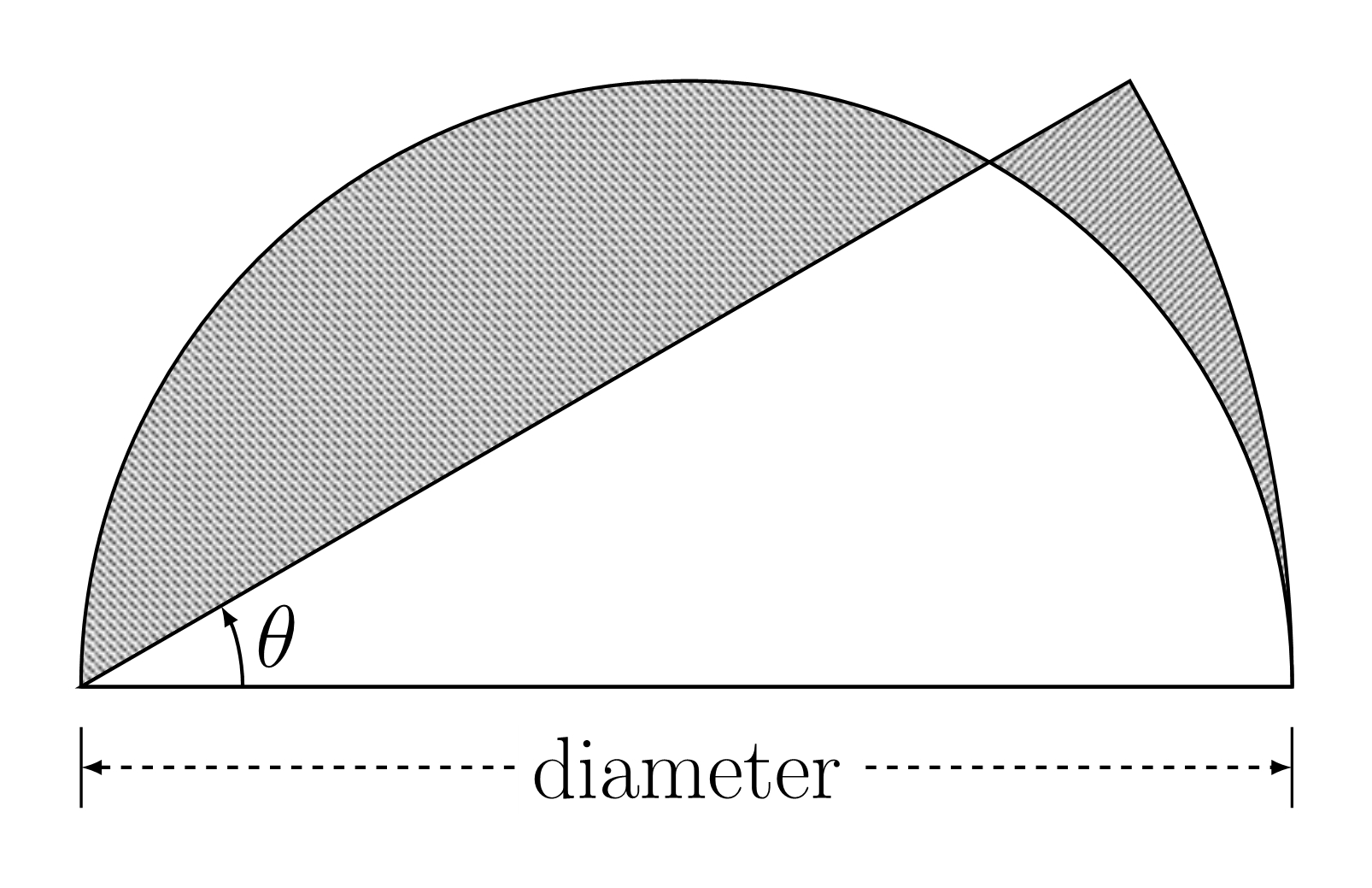# Question 1 of 83

The figure shows a semicircle and a sector overlapping each other. Find the perimeter of the shaded region if $\theta=30^\circ$ and diameter $=$ 14 cm. Take $\pi=\frac{22}{7}$.$43$
$43\frac{1}{3}$
$43\frac{2}{3}$
$44$